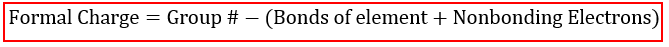# Problem: The thiocyanate ion, NCS-, is linear, with a central carbon atom. Construct all feasible electron-dot diagrams for this ion; then use the concept of formal charge to identify the most probable contributing structures. Display the result using a partial bond representation.

###### FREE Expert Solution

We are being asked to construct all feasible electron-dot diagrams for the thiocyanate ion, SCN- and then use the concept of formal charge to identify the most probable contributing structures.

We are also asked to present the result using a partial bond representation.

First, we will determine the Lewis structure of the given compound using the steps below:

Step 1. Calculate the total number of valence electrons.

Step 2. Determine the central atom in the molecule.

Step 3. Draw the Lewis structure for the molecule and employ the concept of formal charge:Second, is to present the results using partial bond representation.

99% (259 ratings)###### Problem Details

The thiocyanate ion, NCS-, is linear, with a central carbon atom. Construct all feasible electron-dot diagrams for this ion; then use the concept of formal charge to identify the most probable contributing structures. Display the result using a partial bond representation.